C Paleomagnetic statistics and parameter estimation

Appendix CPaleomagnetic statistics and parameter estimation

Chapters 5 and 7 discussed various hysteresis parameters and Chapters 11 and 12 developed the major features of paleomagnetic directional statistics. Here we go over some aspects in greater detail.

C.1 Hysteresis Parameters

A typical hysteresis experiment involves determination of a hysteresis loop and frequently also a back-field curve (see Figure C.1). Processing of the data in the PmagPy software package (see, e.g., Example for hysteresis_magic.py) proceeds as follows:

1. Sometimes the descending and ascending hysteresis loops do not close because of instrument drift (see Figure C.1a). Ordinarily, the experiment should be re-done, but for small differences, we force the loops to close by subtracting the difference, interpolated from the maximum difference at the maximum field (Bmax) to a zero difference at the minimum field (Bmin).
2. After closing the loops, we calculate the best-fit line to the M,B data for the portion within 70% of Bmax, averaging data from both the ascending and descending loops. A difference in the absolute value of the y-intercepts for the ascending and descending loops indicates a vertical offset of the data, which is adjusted such that the two intercepts are equal. The average slope is the high-field susceptibility (χhf), which is subtracted off. The data after these steps are shown as the dashed line in Figure C.1. The maximum magnetization after adjusting for the χhf is the saturation magnetization Ms.

Table C.1: Summary of hysteresis parameters.
 Symbol Method Section Figure χhf high field susceptibility 5.2.2 & 8.5 5.6 &  C.1 Ms saturation magnetization 3.2.2 5.6 &  C.1 Mr saturation remanence 5.2.1 & 7.7 5.6 &  C.1 Hc or μoHc Coercivity 4.1.3 &  5.2.1 5.6 &  C.1 Coercivity of remanence: Hcr ΔM method 5.2.1 C.1 Hcr′ ascending loop intercept method 5.2.1 5.6 Hcr′′ Back-field method 7.7, 7.20 & C.1 Hcr′′′ H1∕2 method 7.7 & 8.4.2 7.20 &  8.9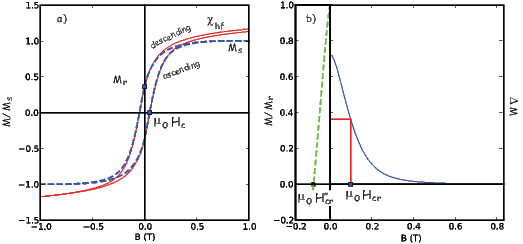Figure C.1: Typical hysteresis experiment. a) Raw data are solid red line. Data are processed (see text) by closing the ascending and descending loops, subtracting the high field slope (χhf) and adjusting such that the y-intercepts are equal (that for the descending loop is labeled Mr). Processed data are dotted blue line. Coercivity (μoHc) is the applied field for which a saturation magnetization (Ms) is reduced to zero. b) Difference between processed ascending and descending loops is the ΔM curve (solid blue line). Back-field IRM data shown normalized by saturation remanence (Mr) – dashed green line. Two methods of estimating coercivity of remanence shown (see text).

3. Coercivity (μoHc) is the field at which M = 0. We estimate this by finding the values of B between which M switches sign for both the ascending and descending loops (after adjustment), calculate a line and evaluate the B for which M = 0. The coercivity is the average of the two estimates.
4. We fit a spline to the adjusted ascending and descending loops and resample the loops at even intervals of B (usually 10 mT intervals). The ΔM curve shown in Figure C.1b is the difference between these two interpolated curves, averaging the data for negative and positive B. The saturation remanence Mr is the value of the ΔM curve at B = 0. The coercivity of remanence (μoHcr in Table C.1) is the field for which ΔM is half the value of Mr. This is the “ΔM” method of coercivity of remanence calculation (see Chapter 5).
5. If there are “back-field” IRM data as in Figure C.1b, the coercivity of remanence can be estimated by finding (through interpolation) the applied field which reduces the saturation remanence (Mr) to zero. This is the “back field” method.

C.2 Directional statistics

Table C.2: Critical values of Ro for a random distribution [Watson, 1956.]
 N 95% 99% N 95% 99% 5 3.50 4.02 13 5.75 6.84 6 3.85 4.48 14 5.98 7.11 7 4.18 4.89 15 6.19 7.36 8 4.48 5.26 16 6.40 7.60 9 4.76 5.61 17 6.60 7.84 10 5.03 5.94 18 6.79 8.08 11 5.29 6.25 19 6.98 8.33 12 5.52 6.55 20 7.17 8.55

C.2.1 Calculation of Watson’s V w

1. Calculate Ri, and ki where i = 1,2 for the two data sets with N1,N2 samples using Equations 11.6 and  11.8.
2. Calculate xij (where j = 1,3 for the three axes) using Equation 11.7.
3. Calculate Xij = Rixij.
4. Find the weighted means for the two data sets: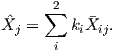5. Calculate the weighted overall resultant vector Rw by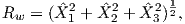and the weighted sum Sw by,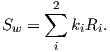6. Finally, Watson’s V w is defined as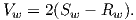C.2.2 Combining lines and planes

1. Calculate M directed lines (two in our case) and N great circles (one in our case) using principal component analysis (see Chapter 9) or Fisher statistics.
2. Assume that the primary direction of magnetization for the samples with great circles lies somewhere along the great circle path (i.e., within the plane).
3. Assume that the set of M directed lines and N unknown directions are drawn from a Fisher distribution.
4. Iteratively search along the great circle paths for directions that maximize the resultant vector R for the M + N directions.
5. Having found the set of N directions that lie along their respective great circles, estimate the mean direction using Equation 11.7 and κ as: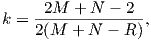The cone of 95% confidence about the mean is given by: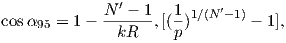where N= M + N∕2 and p = .02

Table C.3: Maximum likelihood estimators of k1,k2 in the Bingham distribution for given eigenvalues ω12. Data from Mardia and Zemroch (1977). Upper (lower) number is k1(k2)
 ω1 0.02 0.04 0.06 0.08 0.10 0.12 0.14 0.16 0.18 0.20 0.22 0.24 0.26 0.28 0.30 0.32 ω2 0.02 -25.55 -25.55 0.04 -25.56 -13.11 -13.09 -13.11 0.06 -25.58 -13.14 -9.043 -8.996 -9.019 -9.043 0.08 -25.6 -13.16 -9.065 -7.035 -6.977 -6.999 -7.020 -7.035 0.10 -25.62 -13.18 -9.080 -7.042 -5.797 -5.760 -5.777 -5.791 -5.798 -5.797 0.12 -25.63 -13.19 -9.087 -7.041 -5.789 -4.917 -4.923 -4.934 -4.941 -4.941 -4.933 -4.917 0.14 -25.64 -13.20 -9.087 -7.033 -5.773 -4.896 -4.231 -4.295 -4.301 -4.301 -4.294 -4.279 -4.258 -4.231 0.16 -25.65 -13.20 -9.081 -7.019 -5.752 -4.868 -4.198 -3.659 -3.796 -3.796 -3.790 -3.777 -3.756 -3.729 -3.697 -3.659 0.18 -25.65 -13.19 -9.068 -6.999 -5.726 -4.836 -4.160 -3.616 -3.160 -3.381 -3.375 -3.363 -3.345 -3.319 -3.287 -3.249 -3.207 -3.160 0.20 -25.64 -13.18 -9.05 -6.974 -5.694 -4.799 -4.118 -3.570 -3.109 -2.709 -3.025 -3.014 -2.997 -2.973 -2.942 -2.905 -2.863 -2.816 -2.765 -2.709 0.22 -25.63 -13.17 -9.027 -6.944 -5.658 -4.757 -4.071 -3.518 -3.053 -2.649 -2.289 -2.712 -2.695 -2.673 -2.644 -2.609 -2.568 -2.521 -2.470 -2.414 -2.354 -2.289 0.24 -25.61 -23.14 -8.999 -6.910 -5.618 -4.711 -4.021 -3.463 -2.993 -2.584 -2.220 -1.888 -2.431 -2.410 -2.382 -2.349 -2.309 -2.263 -2.212 -2.157 -2.097 -2.032 -1.963 -1.888 0.26 -25.59 -13.12 -8.966 -6.870 -5.573 -4.661 -3.965 -3.403 -2.928 -2.515 -2.146 -1.809 -1.497 -2.175 -2.149 -2.117 -2.078 -2.034 -1.984 -1.929 -1.869 -1.805 -1.735 -1.661 -1.582 -1.497 0.28 -25.57 -13.09 -8.928 -6.827 -5.523 -4.606 -3.906 -3.338 -2.859 -2.441 -2.066 -1.724 -1.406 -1.106 -1.939 -1.908 -1.871 -1.828 -1.779 -1.725 -1.665 -1.601 -1.532 -1.458 -1.378 -1.294 -1.203 -1.106 0.30 -25.54 -13.05 -8.886 -6.778 -5.469 -4.547 -3.842 -3.269 -2.785 -2.361 -1.981 -1.634 -1.309 -1.002 -0.708 -1.718 -1.682 -1.641 -1.596 -1.540 -1.481 -1.417 -1.348 -1.274 -1.195 -1.110 -1.020 -0.923 -0.819 -0.708 0.32 -25.50 -13.01 -8.839 -6.725 -5.411 -4.484 -3.773 -3.195 -2.706 -2.277 -1.891 -1.537 -1.206 -0.891 -0.588 -0.292 -1.510 -1.470 -1.423 -1.371 -1.313 -1.250 -1.181 -1.108 -1.028 -0.944 -0.853 -0.756 -0.653 -0.541 -0.421 -0.292 0.34 -25.46 -12.96 -8.788 -6.668 -5.348 -4.415 -3.699 -3.116 -2.621 -2.186 -1.794 -1.433 -1.094 -0.771 -0.459 -0.152 -1.312 -1.267 -1.216 -1.159 -1.096 -1.028 -0.955 -0.876 -0.791 -0.701 -0.604 -0.500 -0.389 -0.269 -0.140 0.000 0.36 -25.42 -12.91 -8.731 -6.606 -5.280 -4.342 -3.620 -3.032 -2.531 -2.089 -1.690 -1.322 -0.974 -0.642 -1.123 -1.073 -1.017 -9.555 -0.887 -0.814 -0.736 -0.651 -0.561 -0.464 -0.360 -0.249 -0.129 0.000 0.38 -25.37 -12.86 -8.670 -6.539 -5.207 -4.263 -3.536 -2.941 -2.434 -1.986 -1.579 -1.202 -0.940 -0.885 -0.824 -0.757 -0.684 -0.606 -0.522 -0.432 -0.335 -0.231 -0.120 0.000 0.40 -25.31 -12.80 -8.604 -6.466 -5.126 -4.179 -3.446 -2.845 -2.330 -1.874 -0.762 -0.702 -0.636 -0.564 -0.486 -0.402 -0.312 -0.215 -0.111 -0.000 0.42 -25.5 -12.73 -8.532 -6.388 -5.045 -4.089 -3.349 -2.741 -0.589 -0.523 -0.452 -0.374 -0.290 -0.200 -0.104 0.000 0.44 -25.19 -12.66 -8.454 -6.305 -4.955 -3.992 -0.418 -0.347 -0.270 -0.186 -0.097 0.000 0.46 -25.12 -12.58 -8.371 -6.215 -0.250 -0.173 -0.090 0.000

C.2.3 Inclination only calculation

We wish to estimate the co-inclination (α = 90 -I) of N Fisher distributed data (αi), the declinations of which are unknown. We define the estimated value of α to be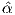. McFadden and Reid showed that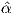is the solution of:which can be solved numerically.

They further define two parameters S and C as: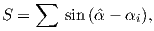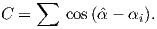An unbiassed approximation for the Fisher parameter κ, k is given by: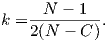The unbiased estimate of the true inclination is: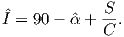Finally, the α95 is estimated by: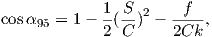where f is the critical value taken from the F distribution (see F-distribution tables in statistics textbooks or online) with 1 and (N-1) degrees of freedom.

C.2.4 Kent 95% confidence ellipse

Kent parameters are calculated by rotating unimodal directions x into the data coordinates xby the transformation: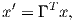(C.1)

where Γ = (γ123), and the columns of Γ are called the constrained eigenvectors of orientation matrix, T (see Appendix A.3.5). The vector γ1 is parallel to the Fisher mean of the data, whereas γ2 and γ3 (the major and minor axes) diagonalize T as much as possible subject to being constrained by γ1 (see Kent, 1982, but note that his x1 corresponds to x3 in conventional paleomagnetic notation). The following parameters may then be computed: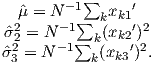(C.2)

As defined here,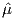= R∕N (R is closely approximated by the equation for R in Chapter 11. Also to good approximation,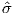22 = τ2, and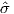32 = τ3, where τi are the eigenvalues of the orientation matrix. The semi-angles ζ95 and η95 subtended by the major and minor axes of the 95% confidence ellipse are given by: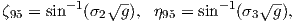(C.3)

where g = -2 ln(0.05)(N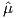2).

The tensor Γ is, to a good approximation, equivalent to V, the eigenvectors of the orientation matrix. Therefore, the eigenvectors of the orientation matrix V give a good estimate for the directions of the semi-angles by: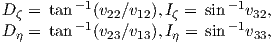(C.4)

where for example the x2 component of the smallest eigenvector V3 is denoted v23.

C.2.5 Bingham 95% confidence parameters

The Bingham distribution is given by: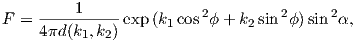where α and ϕ are as in the Kent distribution, k1,k2 are concentration parameters (k1 < k2 < 0) and d(k1,k2) is a constant of normalization given by: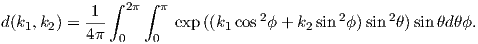To estimate the axes of the Bingham confidence ellipse, we first calculate the eigenparameters of the orientation matrix as for Kent parameters and described in Appendices A.3.5 and  C.2.4. The principle eigenvector V1 of the orientation matrix is associated with the largest eigenvalue τ1. In Bingham (1974), ω1 is the τ3 and ω3 is τ1. In Bingham statistics, the V1 direction is taken as the mean. Beware – it is not always parallel to the Fisher mean of a unimodal set of directions.

The maximum likelihood estimates of k1,k2, the concentration parameters are gotten by first maximizing the log likelihood function: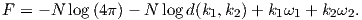These are listed for convenience in Table C.3 as calculated by Mardia and Zemroch (1977). Once these are estimated, the semi-axes of the 95% confidence ellipse around the mean direction V1 are given by: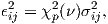where χp2(ν) = 5.99 is the χ2 value for significance (p = .05 for 95% confidence) with ν = 2 degrees of freedom and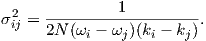Bingham (1974) set k3 = 0, so the semi axes of the confidence ellipse about the principle direction V1, associated with ω3, are therefore: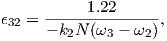and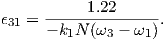Because k1 < k2 < 0, the semi-axes are positive numbers. Please note that here we use the corrected version of Tanaka (1999) as opposed to the more oft-quoted but erroneous treatment of Onstott (1980). Note also that the N is required for σ because we have normalized the ωs to sum to unity for consistency with other eigenvalue problems in this book. The N is missing in the treatment of Tanaka (1999) presumably because the eigenvalues sum to N. Finally, note that these values of ϵ are in radians and must be converted to degrees for most applications.

C.3 Paleointensity statistics

Paleointensity statistics have gotten somewhat out of hand of late. There are a bewildering variety of statistics that are used in the literature, with no consensus as to which ones are essential, which ones are helpful and which ones are irrelevant. This appendix will not help the reader in this regard, but merely attempts to assemble the ones we feel are the most useful.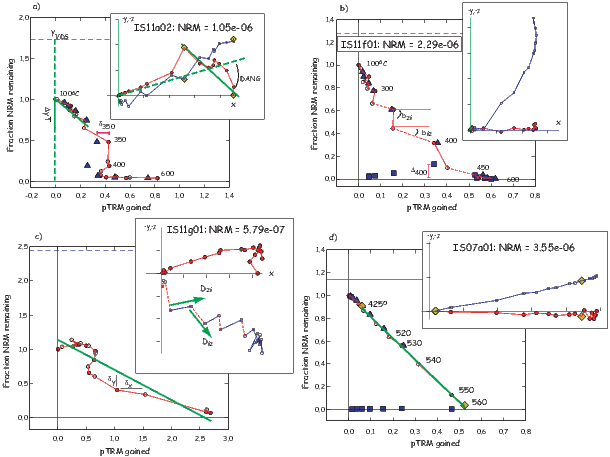Figure C.2: Illustration of paleointensity parameters. Arai plots: The magnitude of the NRM remaining after each step is plotted versus the pTRM gained at each temperature step. Closed symbols are zero-field first followed by in-field steps (ZI) while open symbols are in-field first followed by zero field (IZ). Triangles are pTRM checks and squares are pTRM tail checks. Horizontal dashed lines are the vector difference sum (VDS) of the NRM steps. Vector endpoint plots: Insets are the x,y (solid symbols) and x,z (open symbols) projections of the (unoriented) natural remanence (zero field steps) as it evolves from the initial state (plus signs) to the demagnetized state. The laboratory field was applied along -Z. Diamonds indicate bounding steps for calculations. a) The fvds is the fraction of the component used of the total VDS. The difference between the pTRM check and the original measurement at each step is δTi. The inset shows the deviation angle (DANG) that a component of NRM makes with the origin. The maximum angle of deviation MAD is calculated from the scatter of the points about the best-fit line (solid green line). b) Data exhibit zig-zag behavior diagnostic for significant difference between blocking and unblocking temperatures. The Zig-zag for slopes compares slopes calculated between ZI and IZ steps (bzi) with those connecting IZ and ZI steps biz). The difference between the pTRM tail check and the original measurement at each step is ΔTi. c) β reflects the scatter (δxy) about the best-fit slope (solid green line). The Zig-zag for directions compares those calculated between ZI and IZ steps (Dzi) with those connecting IZ and ZI steps Diz). [Figures from Ben-Yosef et al., 2008.]

1. The Deviation of the ANGle (DANG; Tauxe and Staudigel, 2004; see Chapter 9): The angle that the direction of the NRM component used in the slope calculations calculated as a best-fit line (see Appendix A.3.5) makes with the angle of the line anchoring the center of mass (see Appendix A.3.5) to the origin (see insert to Fig. C.2a).
2. The Maximum Angle of Deviation (MAD; Kirschvink, 1980; see Chapter 9): The scatter about the best-fit line through the NRM steps.
3. We can calculate the best-fit slope (b) for the data on the NRM-pTRM plot and its standard error σ (York, 1966; Coe et al. 1978). The procedure for calculating the best-fit slope, which is the best estimate for the paleofield, is given as follows:

a)Take the N data points that span two temperature steps T1 and T2, the best-fit slope b relating the NRM (yi) and the pTRM (xi) data in a least squares sense (taking into account variations in both x and y is given by: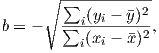(C.5)

where y is the average of all y values and x is the average of all x values.

b) The y-intercept (yo) is given by y - bx.

c) The standard error of the slope σ is: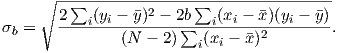(C.6)

4. The “scatter” parameter β: the standard error of the slope σ (assuming uncertainty in both the pTRM and NRM data) over the absolute value of the best-fit slope |b| (Coe et al. 1978).
5. The remanence fraction, f, was defined by Coe et al. (1978) as: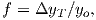where ΔyT is the length of the NRM segment used in the slope calculation (see Figure C.2).
6. The fraction of the total remanence (by vector difference sum), fvds (Tauxe and Staudigel, 2004): While f works well with single component magnetizations as in Fig. C.2d where it reflects the fraction of the total NRM used in the slope calculation, it can be misleading when there are multiple components of remanence as in Fig. C.2a. The values of f for such specimens can be quite high, whereas the fraction of the total NRM is much less. We prefer to use a parameter fvds which is the fraction of the total NRM, estimated by the vector difference sum (VDS; Chapter 9) of the entire zero field demagnetization data. The VDS (see Fig. C.2a) “straightens out” the various components of the NRM by summing up the vector differences at each demagnetization step. fvds is calculated as: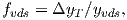where yvds is the vector difference sum of the entire NRM (see Figure C.2a and Chapter 9). This parameter becomes small, if the remanence is multi-component, whereas the original f can be blind to multi-component remanences.

7. The Difference RATio Sum, DRATS: The difference between the original pTRM at a given temperature step (horizontal component of the circles in Fig. C.2) and the pTRM check (horizontal component of the triangles in see Fig. C.2), δi (see Fig. C.2a), can result from experimental noise or from alteration during the experiment. Selkin and Tauxe (2000) normalized the maximum δi value within the region of interest by the length of the hypotenuse of the NRM/pTRM data used in the slope calculation. DRAT is therefore the maximum difference ratio expressed as a percentage. In many cases, it is useful to consider the trend of the pTRM checks as well as their maximum deviations. We follow Tauxe and Staudigel (2004) who used the sum of these differences. We normalize this difference sum by the pTRM acquired by cooling from the maximum temperature step used in the slope calculation to room temperature. This parameter is called the Difference RATio Sum or DRATS. Only pTRM checks at temperatures below the maximum bound are included in the DRATS calculation.
8. Maximum Difference % MD%: The absolute value of the difference between the original NRM measured at a given temperature step (vertical component of the circles in Fig. C.2) and the second zero field step (known as the pTRM tail check) results from some of the pTRM imparted in the laboratory at Ti having unblocking temperatures that are greater than Ti. These differences (Δi; see Fig. C.2b) are plotted as squares. The Maximum Difference, normalized by the VDS of the NRM and expressed as a percentage is the parameter MD%.
9. Zig-Zag Z: In certain specimens, the IZZI protocol leads to rather interesting behavior, described in detail by Yu et al. (2004). The solid symbols in Fig. C.2 are the zerofield-infield (ZI) steps and the intervening steps are the infield-zerofield (IZ) steps (open circles). Alternating the two results in a “zigzag” in some specimens. The zigzag can be in either the Arai diagrams (compare slope of solid versus dashed line segments in Fig. C.2b) or in the orthogonal projections or the zero field vectors (compare directions of solid and dashed line segments in Fig. C.2 c). We therefore can define a parameter Z by testing the difference in either the two sets of slopes or the two sets of directions between the IZ steps and the ZI steps.

To test the significance of the difference between the zero-field IZ directions and those from the ZI zero-field steps, we calculate F-test (Fw) for Watson’s test for common mean (Watson, 1983). The zigzag for directions Zdir is ratio Fw∕F(ν,) where F(ν) is the critical value for F at ν = 2N - 2 degrees of freedom (at the 95% level of confidence).

For the slopes, we calculate the mean and variance of the slopes for the IZ segments (biziz2) and the ZI segments (bzizi2). The parameter tb is the t test for the two means. The zigzag for the slopes Zslope is the ratio tb∕t(ν) where t(ν) is critical value for t with ν = Niz + Nzi - 2 degrees of freedom (from a statistics table).

If the difference between the sets of directions and slopes is less than 2 or both Zslope and Zdir are less than unity, then Z = 0. Otherwise Z is the larger of Zdir and Zslope.

10. The “gap factor” g (Coe et al. 1978) penalizes uneven distribution of data points and is: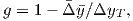where Δy is given by :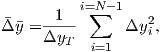and is the weighted mean of he gaps Δyi between the N data points along the selected segment.
11. The Coe quality index q combines the standard error of the slope, the NRM fraction and the gap factors by: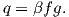As data spacing becomes less uniform, g decreases.

12. A quick and dirty test for the possibility of anisotropy of TRM is to compare the direction of the pTRM acquired in the laboratory field with the direction of the applied field. The angle between these two at the maximum pTRM temperature used in the slope calculation is defined here as γ. If this exceeds more than a few degrees, it is advisable to perform some sort of test for TRM (or ARM) anisotropy.